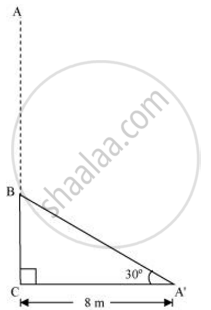Share

# A tree breaks due to storm and the broken part bends so that the top of the tree touches the ground making an angle 30 ° with it - CBSE Class 10 - Mathematics

#### Question

A tree breaks due to storm and the broken part bends so that the top of the tree touches the ground making an angle 30 ° with it. The distance between the foot of the tree to the point where the top touches the ground is 8 m. Find the height of the tree.

#### SolutionLet AC was the original tree. Due to storm, it was broken into two parts. The broken part A'B is making 30° with the ground.

In ΔA'BC

BC/A'C = tan 30º

BC/8 = 1/ sqrt3

BC = (8/sqrt3)m

A'C'/A'B =  cos 30º

8/(A'B) = sqrt3/2

A'B =  ((16)/sqrt3)m

Height of tree = A'B+BC

=(16/sqrt3+8/sqrt3)m = 24/sqrt3 m

= 8sqrt3m

Hence the height of the tree is 8sqrt3 m

Is there an error in this question or solution?

#### APPEARS IN

NCERT Solution for Mathematics Textbook for Class 10 (2019 to Current)
Chapter 9: Some Applications of Trigonometry
Ex. 9.10 | Q: 2 | Page no. 203
NCERT Solution for Mathematics Textbook for Class 10 (2018 to Current)
Chapter 9: Some Applications of Trigonometry
Ex. 9.10 | Q: 2 | Page no. 203

#### Video TutorialsVIEW ALL 

Solution A tree breaks due to storm and the broken part bends so that the top of the tree touches the ground making an angle 30 ° with it Concept: Heights and Distances.
S